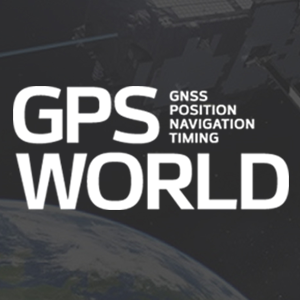# GNSS Spoofing Detection: Guard against automated ground vehicle attacks

February 23, 2023  -### MEASUREMENT MODEL

The full formulation of the measurement model for the tightly coupled GNSS-IMU estimator on which our spoofing detection technique is based is presented in a paper accepted for publication in the Institute of Navigation’s journal NAVIGATION. Below are key developments.

The estimator ingests pairs of double-difference (DD) GNSS observables at each GNSS measurement epoch, with each pair composed of a pseudorange and a carrier-phase measurement.

After linearizing about the a priori state estimate, a measurement model for the innovations vector can be expressed by setting the difference between the measurement vector and its modeled value based on the a priori state estimate equal to the corresponding Jacobians (matrices of partial derivatives), the state estimate error vector, and the integer ambiguity vector.

A short-baseline regime is assumed for the DD measurements, which implies that ionospheric, tropospheric, ephemeris and clock errors are cancelled in the double differencing. The CDGNSS measurement update of the tightly coupled GNSS-IMU estimator can be cast in square-root form for greater numerical robustness and algorithmic clarity. The measurement update can be defined as the process of finding the state estimate error vector and the integer ambiguity vector to minimize the cost function, a term borrowed from economics theory, which is essentially the sum of the squared errors.

The cost function can be decomposed into three terms: a term that can be zeroed for any value of the integer ambiguity vector; a term corresponding to the residual cost of enforcing the integer constraint; and the irreducible cost that can be shown to be equivalent to the normalized innovations associated with the DD pseudorange measurements.

We normalize the vector cost components by using square-root information matrices based on Cholesky factorization followed by the cost decomposition using QR factorization. The equations are then solved to provide float (real number) values for the state estimate error vector and the integer ambiguity vector. Subsequently, the fixed solution is found via an integer least squares (ILS) solver.

### TEST STATISTIC

Key to our spoofing detection statistic is the integer-fixed carrier-phase residual cost value, which also can be thought of as the ILS solution cost. This is equivalent to the second term of the decomposed cost function stated earlier. This is small whenever the carrier-phase measurements are consistent with the prior state estimate and the pseudorange measurements, and with the assumption of integer-valued carrier-phase ambiguities.

It is one of several acceptance test statistics used to decide whether the fixed solution is correct with high probability. It has been incorporated, for example, in a statistic used to detect carrier cycle slips. It can be similarly used to detect false integer fixes, just as with other integer aperture acceptance test statistics, or the lingering effects of conditioning the real-valued part of the state on a previous false fix.

Furthermore, the test statistic provides a highly sensitive statistic for spoofing detection. When no spoofing is present, there is tight agreement between the IMU-propagated a priori state estimate and GNSS data resulting in a small statistic value.

If the vehicle hits a bump in the road, the GNSS antenna phase center will rise by a few centimeters, and the inertial sensor will detect a corresponding acceleration, which will get propagated through to the a priori state. On the other hand, when spoofing is present, a discrepancy between inertial and GNSS data will arise at the carrier-phase level, leading to the statistic being larger than usual.

A windowed sum of test statistic values offers even greater sensitivity to false-fix events at the expense of a longer time-to-detect. To detect spoofing in the tests reported in this article, we used the windowed fixed-ambiguity residual cost (WFARC). This is calculated over a moving window of fixed length of past GNSS measurement epochs. We used a window length of 10 past GNSS measurement epochs (amounting to a window of 2 seconds).

If the filter is consistent and the integer ambiguities are correctly resolved, then WFARC should be approximately χ2-distributed with the degrees of freedom related to the number of DD carrier-phase measurements. This distribution is approximate due to the “integer-folding” effect: large phase residuals are not possible because of integer-cycle phase wrapping. A statistical consistency test can be performed by choosing a desired false-alarm rate and declaring a false fix if WFARC is greater than a specified threshold calculated by evaluating the inverse cumulative distribution function of χ2 at the false-alarm rate.### About the Author: Zachary Clements

Zachary Clements (BS, Electrical Engineering, Clemson University) is a graduate student in the Department of Aerospace Engineering, and Engineering Mechanics at The University of Texas (UT) at Austin, and a member of the UT Radionavigation Laboratory.### About the Author: James Yoder

James Yoder (BS, Electrical Engineering, MS, Aerospace Engineering, UT Austin) is a guidance, navigation and control engineer at SpaceX and an alumnus of the UT Radionavigation Laboratory.### About the Author: Todd E. Humphreys

Todd Humphreys (BS, MS, Electrical Engineering, Utah State University; PhD, Aerospace Engineering, Cornell University) is a professor in the Department of Aerospace Engineering and Engineering Mechanics at UT Austin, where he directs the Radionavigation Laboratory.